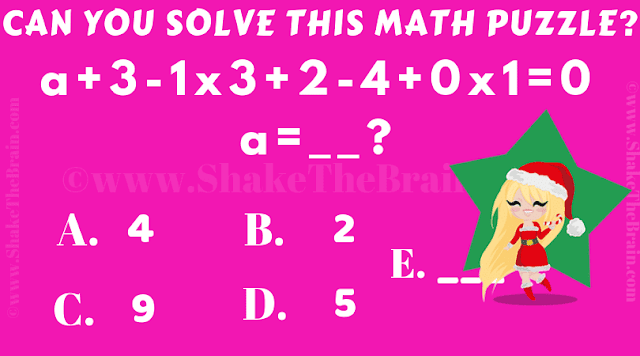## Sunday, April 21, 2019

If you are a student, then it is time to test your Mathematical knowledge and skills with this simple Maths Test. In this Puzzle Picture, one Mathematical equation is given. Can you solve this equation for variable a?Can you solve this?

Answer of this "Mathematical Puzzle for Teens",  can be viewed by clicking on the button. Please do give your best try before looking at the answer.

1.1.